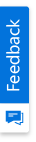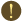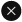We use cookies to give you the best experience on our website. If you continue to browse, then you agree to our privacy policy and cookie policy.Unfortunately, activation email could not send to your email. Please try again.# How to use size,position,fittocell arguments in template markers using XlsIO?

Platform: WinForms |
Control: XlsIO
Tags:

This article explains how to use size, position and fittocell argument in template markers using XlsIO.

What is size argument?

The argument size specified in template marker applies the image to the specified size inside the cell. Height parameter is optional. Value of width is applied when height is not specified.

Syntax

 %.;size:width,height

Note:

By default, the image width and height is set to 50.

Steps to use size argument

Create a workbook template with markers in it. Here, Employee is the marker variable referred to a class object, followed by its properties separated by dot (.).

```//Adding markers dynamically with the argument, 'size'
worksheet["A4"].Text = "%Employee.Image; size:60,80";
worksheet["B4"].Text = "%Employee.Name";
worksheet["C4"].Text = "%Employee.Id";
worksheet["D4"].Text = "%Employee.Age";
```

The below screenshot shows how the markers are applied in workbook template.Size Argument

The below screenshot shows the output document generated using template markers with size argument.Size Argument

What is position argument?

The argument position specified in template marker applies the image to the specified position inside the cell.

The possible horizontal positions are listed below,

• Left
• Center
• Right

The possible vertical positions are listed below,

• Top
• Middle
• Bottom

Syntax to mention the image horizontal and vertical positions

 %.;position:top-right

Syntax to mention the image horizontal position

 %.;position:right

Syntax to mention the image vertical position

 %.;position:top

Note:

By default, the horizontal position is top and vertical position is left.

Steps to use position argument

Create a workbook template with markers in it. Here, Employee is the marker variable referred to a class object, followed by its properties separated by dot (.).

```//Adding markers dynamically with the argument, 'size'
worksheet["A4"].Text = "%Employee.Image;position:right";
worksheet["B4"].Text = "%Employee.Name";
worksheet["C4"].Text = "%Employee.Id";
worksheet["D4"].Text = "%Employee.Age";
```

The below screenshot shows how the markers are applied in workbook template.Position Argument

The below screenshot shows the output document generated using template markers with position argument.Position Argument

How to use size and position argument at same time?

The arguments position and size can be used as a combination to specify the size for the image and to specify the position of the image.

Syntax

 %.;size:width,height;position:right

Steps to use size and position argument

Create a workbook template with markers in it. Here, Employee is the marker variable referred to a class object, followed by its properties separated by dot (.).

```//Adding markers dynamically with the argument, 'size' and ‘position’
worksheet["A4"].Text = "%Employee.Image;size:40;position:center";
worksheet["B4"].Text = "%Employee.Name";
worksheet["C4"].Text = "%Employee.Id";
worksheet["D4"].Text = "%Employee.Age";
```

The below screenshot shows how the markers are applied in workbook template.Size and Position Arguments

The below screenshot shows the output document generated using template markers with size and position argument.Size and Position Arguments

What is fit to cell argument?

The argument position specified in template marker applies the image with cell’s height and width.

Syntax

 %.;fittocell

Steps to fittocell argument

Create a workbook template with markers in it. Here, Employee is the marker variable referred to a class object, followed by its properties separated by dot (.).

```//Adding markers dynamically with the argument, 'fittocell'
worksheet["A4"].Text = "%Employee.Image;fittocell”;
worksheet["B4"].Text = "%Employee.Name";
worksheet["C4"].Text = "%Employee.Id";
worksheet["D4"].Text = "%Employee.Age";
```

The below screenshot shows how the markers are applied in workbook template.Fit to cell Argument

The below screenshot shows the output document generated using template markers with fittocell argument.Fit to cell Argument

Steps to use the template marker processor

1. Create template marker processor.

```//Create template marker processor
ITemplateMarkersProcessor marker = workbook.CreateTemplateMarkersProcessor();
```

1. Add a variable name that is equal to the class object specified in the markers added into worksheet.

```//Add marker variable
```

1. Apply markers.

```//Apply markers
marker.ApplyMarkers();
```

The following C#/VB.NET complete code snippet shows how to use template marker with size,position and fittocell argument in XlsIO.

```Syncfusion.XlsIO;
using System.Collections.Generic;
using System.IO;

namespace TemplateMarker
{
public class Employee
{
private byte[] m_image;
private string m_name;
private int m_id;
private int m_age;

public byte[] Image
{
get
{
return m_image;
}
set
{
m_image = value;
}
}
public string Name
{
get
{
return m_name;
}

set
{
m_name = value;
}
}
public int Id
{
get
{
return m_id;
}

set
{
m_id = value;
}
}
public int Age
{
get
{
return m_age;
}

set
{
m_age = value;
}
}
}
}
public static List<Employee> GetEmployeeDetails()
{

List<Employee> employeeList = new List<Employee>();
Employee emp = new Employee();
emp.Image = image1;
emp.Name = "Andy Bernard";
emp.Id = 1011;
emp.Age = 35;

emp = new Employee();
emp.Image = image2;
emp.Name = "Karen Fillippelli";
emp.Id = 1012;
emp.Age = 26;

emp = new Employee();
emp.Image = image3;
emp.Name = "Patricia Mckenna";
emp.Id = 1013;
emp.Age = 28;

return employeeList;
}
private void btnCreate_Click(object sender, System.EventArgs e)
{
ExcelEngine excelEngine = new ExcelEngine();

IApplication application = excelEngine.Excel;
IWorkbook workbook = application.Workbooks.Create(1);
IWorksheet worksheet = workbook.Worksheets;

worksheet["A3"].Text = "Image";
worksheet["B3"].Text = "Name";
worksheet["C3"].Text = "Id";
worksheet["D3"].Text = "Age";

worksheet["A3:D3"].CellStyle.Font.Bold = true;

worksheet["B4"].Text = "%Employee.Name";
worksheet["C4"].Text = "%Employee.Id";
worksheet["D4"].Text = "%Employee.Age";

string fileName = "";
string outputPath = "";

if (imageSize.Checked)
{
fileName = "ImageSizeOnly.xlsx";
//Adding markers dynamically with the argument, 'size'
worksheet["A1"].Text = "\"Size\" Argument";
worksheet["A4"].Text = "%Employee.Image;size:60,80";
}
else if (imagePos.Checked)
{
fileName = "ImageWithPosition.xlsx";
//Adding markers dynamically with the argument, 'position'
worksheet["A1"].Text = "\"Position\" Argument";
worksheet["A4"].Text = "%Employee.Image;position:right";
}
else if (imageSizePos.Checked)
{
fileName = "ImageWithSizeAndPosition.xlsx";
//Adding markers dynamically with the argument, 'size and position'
worksheet["A1"].Text = "\"Size and Position\" Arguments";
worksheet["A4"].Text = "%Employee.Image;size:40;position:center";
}
else if (imageFit.Checked)
{
fileName = "ImageFitToCell.xlsx";
//Adding markers dynamically with the argument, 'fit to cell'
worksheet["A1"].Text = "\"Fit to cell\" Argument";
worksheet["A4"].Text = "%Employee.Image;fittocell";
}

//Create template marker processor
ITemplateMarkersProcessor marker = workbook.CreateTemplateMarkersProcessor();

//Apply markers
marker.ApplyMarkers();

outputPath = GetFullOutputPath(fileName);
worksheet["B1:D10"].AutofitColumns();

//Saving and Closing the workbook.
workbook.SaveAs(outputPath);
workbook.Close();
excelEngine.Dispose();
}
```

```Public Class Employee

Private m_image As Byte()
Private m_name As String
Private m_id As Integer
Private m_age As Integer

Public Property Image As Byte()
Get
Return m_image
End Get

Set(ByVal value As Byte())
m_image = value
End Set
End Property

Public Property Name As String
Get
Return m_name
End Get

Set(ByVal value As String)
m_name = value
End Set
End Property

Public Property Id As Integer
Get
Return m_id
End Get

Set(ByVal value As Integer)
m_id = value
End Set
End Property

Public Property Age As Integer
Get
Return m_age
End Get

Set(ByVal value As Integer)
m_age = value
End Set
End Property
End Class

Public Function GetEmployeeDetails() As List(Of Employee)

Dim image1 As Byte() = File.ReadAllBytes(GetFullTemplatePath("Man1.png"))
Dim image2 As Byte() = File.ReadAllBytes(GetFullTemplatePath("Man2.png"))
Dim image3 As Byte() = File.ReadAllBytes(GetFullTemplatePath("Woman1.png"))

Dim employeeList As List(Of Employee) = New List(Of Employee)()
Dim emp As Employee = New Employee()
emp.Image = image1
emp.Name = "Andy Bernard"
emp.Id = 1011
emp.Age = 35

emp = New Employee()
emp.Image = image2
emp.Name = "Karen Fillippelli"
emp.Id = 1012
emp.Age = 26

emp = New Employee()
emp.Image = image3
emp.Name = "Patricia Mckenna"
emp.Id = 1013
emp.Age = 28

Return employeeList
End Function

Private Sub btnCreate_Click1(ByVal sender As Object, ByVal e As System.EventArgs) Handles btnCreate.Click

Dim excelEngine As ExcelEngine = New ExcelEngine()
Dim application As IApplication = excelEngine.Excel

Dim workbook As IWorkbook = application.Workbooks.Create(1)
Dim worksheet As IWorksheet = workbook.Worksheets(0)

worksheet("A3").Text = "Image"
worksheet("B3").Text = "Name"
worksheet("C3").Text = "Id"
worksheet("D3").Text = "Age"

worksheet("A3:D3").CellStyle.Font.Bold = True

worksheet("B4").Text = "%Employee.Name"
worksheet("C4").Text = "%Employee.Id"
worksheet("D4").Text = "%Employee.Age"

Dim fileName As String = ""
Dim outputPath As String = ""

If imageSize.Checked Then
fileName = "ImageSizeOnly.xlsx"
'Adding markers dynamically with the argument 'size'
worksheet("A1").Text = """Size"" Argument"
worksheet("A4").Text = "%Employee.Image;size:60,80"

ElseIf imagePos.Checked Then
fileName = "ImageWithPosition.xlsx"
'Adding markers dynamically with the argument 'position'
worksheet("A1").Text = """Position"" Argument"
worksheet("A4").Text = "%Employee.Image;position:right"

ElseIf imageSizePos.Checked Then
fileName = "ImageWithSizeAndPosition.xlsx"
'Adding markers dynamically with the argument 'size and position'
worksheet("A1").Text = """Size and Position"" Arguments"
worksheet("A4").Text = "%Employee.Image;size:40;position:center"

ElseIf imageFit.Checked Then
fileName = "ImageFitToCell.xlsx"
'Adding markers dynamically with the argument 'fittocell'
worksheet("A1").Text = """Fit to cell"" Argument"
worksheet("A4").Text = "%Employee.Image;fittocell"
End If

'Create template marker processor
Dim marker As ITemplateMarkersProcessor = workbook.CreateTemplateMarkersProcessor()

'Apply markers
marker.ApplyMarkers()

outputPath = GetFullOutputPath(fileName)
worksheet("B1:D10").AutofitColumns()

'Saving and closing the workbook
workbook.SaveAs(outputPath)
workbook.Close()
excelEngine.Dispose()

End Sub
```

2X faster development

The ultimate WinForms UI toolkit to boost your development speed.You are using an outdated version of Internet Explorer that may not display all features of this and other websites. Upgrade to Internet Explorer 8 or newer for a better experience.